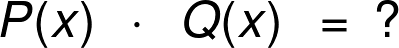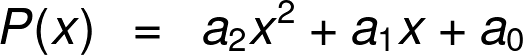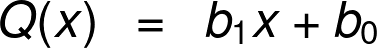# Multiplying Polynomials Calculator

Created by Maciej Kowalski, PhD candidate
Reviewed by Steven Wooding
Last updated: Sep 28, 2022

Welcome to Omni's multiplying polynomials calculator, where we'll study how to find the product of two polynomial expressions. In essence, the most important part here is multiplying monomials, which is what we start with. Then multiplying a polynomial by a monomial means simply taking each term (i.e., each monomial) of the first and multiplying it by the latter expression. Finally, multiplication of polynomials boils down to multiplying the first polynomial by each term of the second.

Don't worry if the above made little sense; we'll go through it all step by step in the sections below. So without further ado, let's jump down and study the topic and Omni's polynomial multiplication calculator.

## Polynomials, monomials, binomials

When we first meet mathematics, we learn about different kinds of numbers from integers, through fractions and decimals, to some mysterious values such as the π that we use when dealing with circles. They teach us how to add them, how to raise them to powers, etc. It's all nice and simple.

However, not all things in life are known from the start. Sometimes we know that there is some number, but we don't know its value. At that moment, mathematics gets involved and denote these values using variables - symbols (usually letters) that represent some objects (usually numbers). If these values satisfy some property or are a part of some large expression, we can describe these connections using formulas or functions. A polynomial is a special case of those.

A polynomial is an algebraic expression that involves numbers and variables in non-negative integer powers. To be precise, they are sums (the same as we work with in our addition calculator), whose every term is a product of numbers and variables in non-negative integer powers (we don't allow negative or fraction exponents). Each summand here is called a monomial (the prefix "mono-" meaning "single"). For instance:

• 2x,

• (-3) * z³ * 0.5,

• πr²,

• a²⁰²⁰,

• ½ * 0.7654321 * 1, and

• k * l * (-7x) * k²,

are all monomials. Note that they don't necessarily involve letters (i.e., variables) and that we can often solve the expression to look much simpler.

A binomial is a polynomial that has two terms (the prefix "bi-" meaning "two"), i.e., it's a sum of two monomials. As such, among the following examples:

• x + 2y,

• a² + 2ab + b,

• n³ - 0.7n + ⅜, and

• 1 + 3 + x⁵ - x⁷ + 19x⁵,

only the first expression is a binomial; the rest are all polynomials.

In fact, we can use many of the operations that we know from arithmetics and apply them to polynomials. For instance, we can add them, divide them (read more about it in our polynomial division calculator), raise them to some power, but most importantly, we can multiply them, which is why we are gathered here today.

However, before introducing the multiplication of polynomials in full generality, let's first analyze the most straightforward case - multiplying monomials.

What does a polynomial look like? Check our polynomial graphing calculator and find out!

## Multiplying monomials

The most basic rule for multiplying monomials is that numbers go with numbers and letters go with letters. Before we explain that precisely, let's have a few examples which will visualize what we say:

• (-3) * z³ * 0.5 = -1.5z³,

• ½ * 0.7654321 * 1 = 0.38271605, and

• k * l * (-7x) * k² = -7k³lx.

Note that these are the monomials from the above section. In particular, that means a product of monomials is again a monomial.

Multiplying monomials boils down to the following set of instructions: multiply all the numbers in the product and then write the letters in alphabetical order. The variables get a power that comes from all occurrences of that letter. For example, in the last expression above, we got the third power of k since we had three k-s in the product to the left (the second one is squared, which alone gives two copies of k). Alternatively, we can state the last sentence differently: the power on the right side is the sum of powers of that variable on the left side.

Alright, we have the first step behind us, and arguably, it wasn't all that bad. Let's try our luck with the next: multiplying a polynomial by a monomial.

## Multiplying a polynomial by a monomial

Fortunately, multiplying a polynomial by a monomial is almost the same thing as multiplying monomials; we simply do it several times.

The instruction is as follows: multiply the monomial by each term (i.e., each monomial) of the polynomial and add it all together. Again, to visualize what we're saying here, let's mention a few examples. Remember that here the initial expressions are in their simplified form, to begin with:

(2 + z + xy) * (-1.5z³)

= 2 * (-1.5z³) + z * (-1.5z³) + xy * (-1.5z³)

= -3z³ - 1.5z⁴ - 1.5xyz³,

and

(100 + 1000a - 10000a³) * 0.38271605

= 100 * 0.38271605 + 1000a * 0.38271605 + (-10000a³) * 0.38271605

= 38.271605 + 382.71605a - 3827.1605a³,

and

(kx + 0.2x³ - 2kl²x) * (-7k³lx)

= kx * (-7k³lx) + 0.2x³ * (-7k³lx) + (-2kl²x) * (-7k³lx)

= -7k⁴lx² - 1.4k³lx⁴ + 14k⁴l³x².

Note how in the last two examples, we had a term with a minus in the initial polynomial. It's vital to remember about the sign in the multiplication of polynomials, and that's why these terms, later on, appeared in brackets with - in front.

That concludes the part on multiplying a polynomial by a monomial. With that information at hand, we're ready to see how to multiply polynomials in general.

## Multiplication of polynomials

As a matter of fact, multiplication of polynomials is just multiplying a polynomial by a monomial, but done several times. The latter, in turn, is simply multiplying monomials several times. And this is how we complete the circle and essentially get back to the information in .

To be precise, multiplication of polynomials means taking one of the expressions and multiplying each of its summands by the other polynomial according to the rules from . As a simple case, let's try multiplying binomials (i.e., polynomials with two terms) first.

Symbolically, we can describe multiplying binomials in the following way:

(A + B) * (C + D)
= A * (C + D) + B * (C + D)
= A*C + A*D + B*C + B*D,

where A, B, C, and D are some monomial expressions. However, multiplication is commutative so we can alternatively consider consecutive terms in the second bracket. That would give:

(A + B) * (C + D)
= (A + B) * C + (A + B) * D
= A*C + B*C + A*D + B*D,

which is the same as above (this time, because addition is commutative).

💡 Multiplying two binomials you get a trinomial, and the reverse operation is called trinomial factorization. If the two binomials were identical, you've obtained a perfect square trinomial. Read more about it on our factoring trinomials calculator and perfect square trinomial calculator!

However, if, say, the first bracket had three terms instead of two, the formula for multiplication of such polynomials would look something like this:

(A + B + C) * (D + E)
= A * (D + E) + B * (D + E) + C * (D + E)
= A*D + A*E + B*D + B*E + C*D + C*E,

or alternatively,

(A + B + C) * (D + E)
= (A + B + C) * D + (A + B + C) * E
= A*D + B*D + C*D + A*E + B*E + C*E.

The two sums are precisely the same, and it's your choice which to use. Nevertheless, you can already see that we get more terms than when multiplying binomials. And you can easily imagine how it'd be even more in case of multiplication of polynomials with even more summands.

Since the thing got a bit lengthy, let's take this opportunity to introduce you to Omni's polynomial multiplication calculator by using the tool along doing the calculations ourselves.

## Example: using the multiplying polynomials calculator

Before we begin, note that Omni's polynomial multiplication calculator only deals with expressions with a single variable. That means the two polynomials in question can only have one letter in them. That being said, we use x in our tool, but you can think of it as any letter you like.

Let's take the following two expressions: x⁴ - 3x² + 2x + 4 and -0.5x² + x - 2. Firstly, we show how to multiply the polynomials using the multiplying polynomials calculator.

At the top of our tool, you see a symbolic expression P(x) * Q(x) = ?, which tells us that the calculator denotes the two polynomials as P(x) and Q(x). Below the formula, we see two areas with variables corresponding to them. First, we need to input the degrees of the polynomials, i.e., the highest power in each of them.

Looking back at the expressions, we see that the terms with the highest exponents are x⁴ and -0.5x², so the degrees are 4 and 2, respectively. Therefore, we input 4 under "Degree" in the area concerning that P(x), and 2 in that of Q(x), which triggers symbolic representations of the two with the notation used underneath.

We see that P(x) = a₄x⁴ + a₃x³ + a₂x² + a₁x + a₀ and Q(x) = b₂x² + b₁x + b₀, so we input:

a₄ = 1, a₃ = 0, a₂ = -3, a₁ = 2, a₀ = 4, and

b₂ = -0.5, b₁ = 1, b₀ = -2.

Note that a₄ = 1 and b₁ = 1 even though we had no number in front of the corresponding powers of x. That is because, by convention, we don't write the coefficient if it's equal to 1 (similarly to how we don't write the ² in square roots). Also, the entries for a₂ and b₂ need to have a minus since the polynomials have minuses in those places. Lastly, observe that a₃ = 0, because we have no x³ in the first polynomial, which means that its coefficient is equal to 0.

The moment we input the last number, the multiplying polynomials calculator will spit out the answer under all the variable fields. However, before we reveal the solution, let's see how to multiply the polynomials ourselves.

As mentioned in , we need to choose one of the two polynomials which we'll decompose into summands, which we'll in turn multiply by the other polynomial according to the rules from . Please observe that the second expression has fewer terms (three instead of four in the first), so let us choose that one to decompose.

(x⁴ - 3x² + 2x + 4) * (-0.5x² + x - 2)

= (x⁴ - 3x² + 2x + 4) * (-0.5x²) + (x⁴ - 3x² + 2x + 4) * x + (x⁴ - 3x² + 2x + 4) * (-2)

= -0.5x⁶ + 1.5x⁴ - x³ - 2x² + x⁵ - 3x³ + 2x² + 4x - 2x⁴ + 6x² - 4x - 8.

However, note that this expression can be simplified further since there are terms with the same power of x. Therefore, simple calculations give:

(x⁴ - 3x² + 2x + 4) * (-0.5x² + x - 2)

= -0.5x⁶ + 1.5x⁴ - x³ - 2x² + x⁵ - 3x³ + 2x² + 4x - 2x⁴ + 6x² - 4x - 8

= -0.5x⁶ + x⁵ - 0.5x⁴ - 4x³ + 6x² - 8,

which is precisely what the multiplying polynomials calculator shows.

Arguably, the computations we did weren't all that difficult, but it's still nice of Omni's polynomial multiplication calculator to save us all that hassle. And time, don't forget the time! After all, they say it's money, don't they?

🙋 You should also check our adding and subtracting polynomials calculator to make sure you understand how to deal with any problem regarding polynomials.

Maciej Kowalski, PhD candidatePolynomial P(x)
Degree
2a₂
a₁
a₀
Polynomial Q(x)
Degree
1b₁
b₀
People also viewed…

### Condition number

The condition number calculator finds the condition number of a matrix. It supports the use of popular matrix norms and can find a 2×2 or 3×3 matrix's condition number.

### Ellipse standard form

In our ellipse standard form calculator, you can determine the standard form of an ellipse by inputting its vertices.

### Free fall

Our free fall calculator can find the velocity of a falling object and the height it drops from.

### Humans vs vampires

Vampire apocalypse calculator shows what would happen if vampires were among us using the predator - prey model.